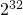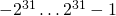# What do +, – and * with Integer do?

When using integers in C, Java or Scala, we often use what is called int.

It is presented to us as the default.

And it is extremely fast.

Ruby uses by default arbitrary length integers.

But what do +, – and * mean?

We can rebuild them, in Ruby, kind of artificially restrict the integers to what we have in other programming langauges as int:

``` MODULUS = 0x100000000; LIMIT   =  0x80000000;```

``` def normalize(x)   r = x % MODULUS;   if (r < -LIMIT) then     return r + MODULUS;   elsif (r >= LIMIT)      return r - MODULUS;   else     return r;   end end def intPlus(x, y)   normalize(x+y); end def intMinus(x, y)   normalize(x-y); end def intTimes(x, y)   normalize(x*y); end ```

```x = 0x7fffffff; y = intPlus(x, x); z = intPlus(x, x); puts("x=#{x} y=#{y} z=#{z}"); ```

What is the outcome?

Exactly what you get when doing 32-Bit-Ints in C, Java, Scala or C#:
``` x=2147483647 y=-2 z=-2 ```

int is always calculated modulo a power of two, usually. That is the
`x % MODULUS ` in `normalize()`. The Rest of the function is just for normalizing the result to the range.

So we silently get this kind of result when an overflow situation occurs, without any notice.
The overflow is not trivial to discover, but it can be done.
For addition I have described how to do it.